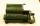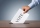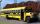Mistake

Nicol mistake when calculate in school. Instead of add number 20 subtract it.

What is the difference between the result and the right result?

Result

x =  -40

Solution:Leave us a comment of example and its solution (i.e. if it is still somewhat unclear...):Be the first to comment!To solve this example are needed these knowledge from mathematics:

Do you have a linear equation or system of equations and looking for its solution? Or do you have quadratic equation?

Next similar examples:

1. I think numberI think number.When I add 841 to it and subtract 157, I get a number that is 22 greater than 996. What number I thinking?
2. Flood waterFlood waters in some US village meant that the homes had to evacuate 364 people. 50 of them stayed at elementary schools, 59 them slept with their friends and others went to relatives. How many people have gone to relatives?
3. Roman numerals 2-Subtract up the number written in Roman numerals. Write the results as Roman numbers.
4. What is missing (1000)What number is to add to get 1000?
5. Roman numerals 2+Add up the number writtens in Roman numerals. Write the results as a roman numbers.
6. Roman numerals +Add up the number writtens in Roman numerals. Write the results as a decimal number.
7. Seven timesWhich number seven times is just as higher as 27, how much is smaller than 29?
8. Number unknownAdela thought the two-digit number, she added it to its ten times and got 407. What number does she think?
9. IntegersMay be the sum of two integers less than their difference?
10. Voting400 employees cast their votes in a board member election that has only 2 candidates. 120 people vote for candidate A, while half of the remaining voters abstain. How many votes does candidate B receive?
11. Write decimalsWrite in the decimal system the short and advanced form of these numbers: a) four thousand seventy-nine b) five hundred and one thousand six hundred and ten c) nine million twenty-six
12. Two numbers 6Fill two natural numbers a, b: 7 + blank- blank = 5
13. Roman numeralsWrite numbers written in Roman numerals as decimal.
14. SummandOne of the summands is 145. The second is 10 more. Determine the sum of the summands.
15. DriveAt the bus stop 8 people take out and 10 people take into bus. Next stop 6 take out and 5 take in. On the third stop 6 take out and 3 take in. The bus traveled further with 39 people. How many passengers were originally at the bus?
16. Eq1Solve equation: 4(a-3)=3(2a-5)
17. If-then equationIf 5x - 17 = -x + 7, then x =